Three equal resistances each of value R are joined as shown in the figure. The equivalent resistance between M and N is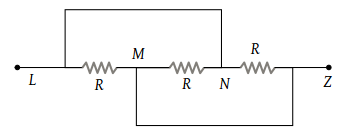(1) R

(2) 2R

(3) $\frac{R}{2}$

(4) $\frac{R}{3}$

Concept Questions :-

Combination of resistors
High Yielding Test Series + Question Bank - NEET 2020

Difficulty Level:

The equivalent resistance between points A and B of an infinite network of resistances each of 1 Ω connected as shown, is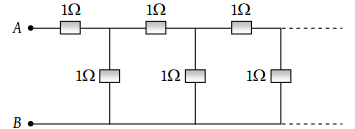(1) Infinite

(2) 2 Ω

(3) $\frac{1+\sqrt{5}}{2}\Omega$

(4) Zero

Concept Questions :-

Combination of resistors
High Yielding Test Series + Question Bank - NEET 2020

Difficulty Level:

In the circuit shown, the point ‘B’ is earthed. The potential at the point ‘A’ is :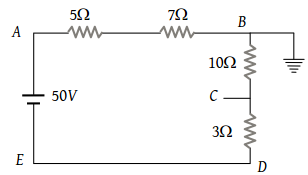(1) 14 V

(2) 24 V

(3) 26 V

(4) 50 V

Concept Questions :-

Combination of resistors
High Yielding Test Series + Question Bank - NEET 2020

Difficulty Level:

In the circuit shown below, the cell has an e.m.f. of 10 V and internal resistance of 1 ohm. The other resistances are shown in the figure. The potential difference ${V}_{A}-{V}_{B}$ is :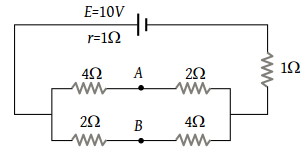(1) 6 V

(2) 4 V

(3) 2 V

(4) –2 V

Concept Questions :-

Combination of resistors
High Yielding Test Series + Question Bank - NEET 2020

Difficulty Level:

A wire of resistance R is cut into ‘n’ equal parts. These parts are then connected in parallel. The equivalent resistance of the combination will be :

(1) nR

(2) $\frac{R}{n}$

(3) $\frac{n}{R}$

(4) $\frac{R}{{n}^{2}}$

Concept Questions :-

Combination of resistors
High Yielding Test Series + Question Bank - NEET 2020

Difficulty Level:

The resistance between the terminal points A and B of the given infinitely long circuit will be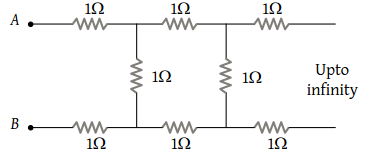(1) $\left(\sqrt{3}-1\right)$

(2) $\left(1-\sqrt{3}\right)$

(3) $\left(1+\sqrt{3}\right)$

(4) $\left(2+\sqrt{3}\right)$

Concept Questions :-

Combination of resistors
High Yielding Test Series + Question Bank - NEET 2020

Difficulty Level:

The current in the given circuit is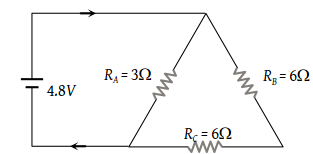(1) 8.31 A

(2) 6.82 A

(3) 4.92 A

(4) 2 A

Concept Questions :-

Combination of resistors
High Yielding Test Series + Question Bank - NEET 2020

Difficulty Level:

What is the current (i) in the circuit as shown in figure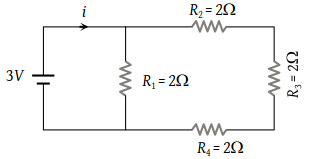(1) 2 A

(2) 1.2 A

(3) 1 A

(4) 0.5 A

Concept Questions :-

Combination of resistors
High Yielding Test Series + Question Bank - NEET 2020

Difficulty Level:

n equal resistors are first connected in series and then connected in parallel. What is the ratio of the maximum to the minimum resistance

(1) n

(2) $\frac{1}{{n}^{2}}$

(3) n2

(4) $\frac{1}{n}$

Concept Questions :-

Combination of resistors
High Yielding Test Series + Question Bank - NEET 2020

Difficulty Level:

In the figure, current through the 3 Ω resistor is 0.8 ampere, then potential drop through 4 Ω resistor is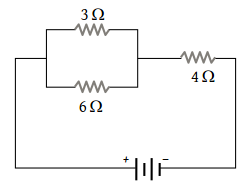(1) 9.6 V

(2) 2.6 V

(3) 4.8 V

(4) 1.2 V

Concept Questions :-

Combination of resistors